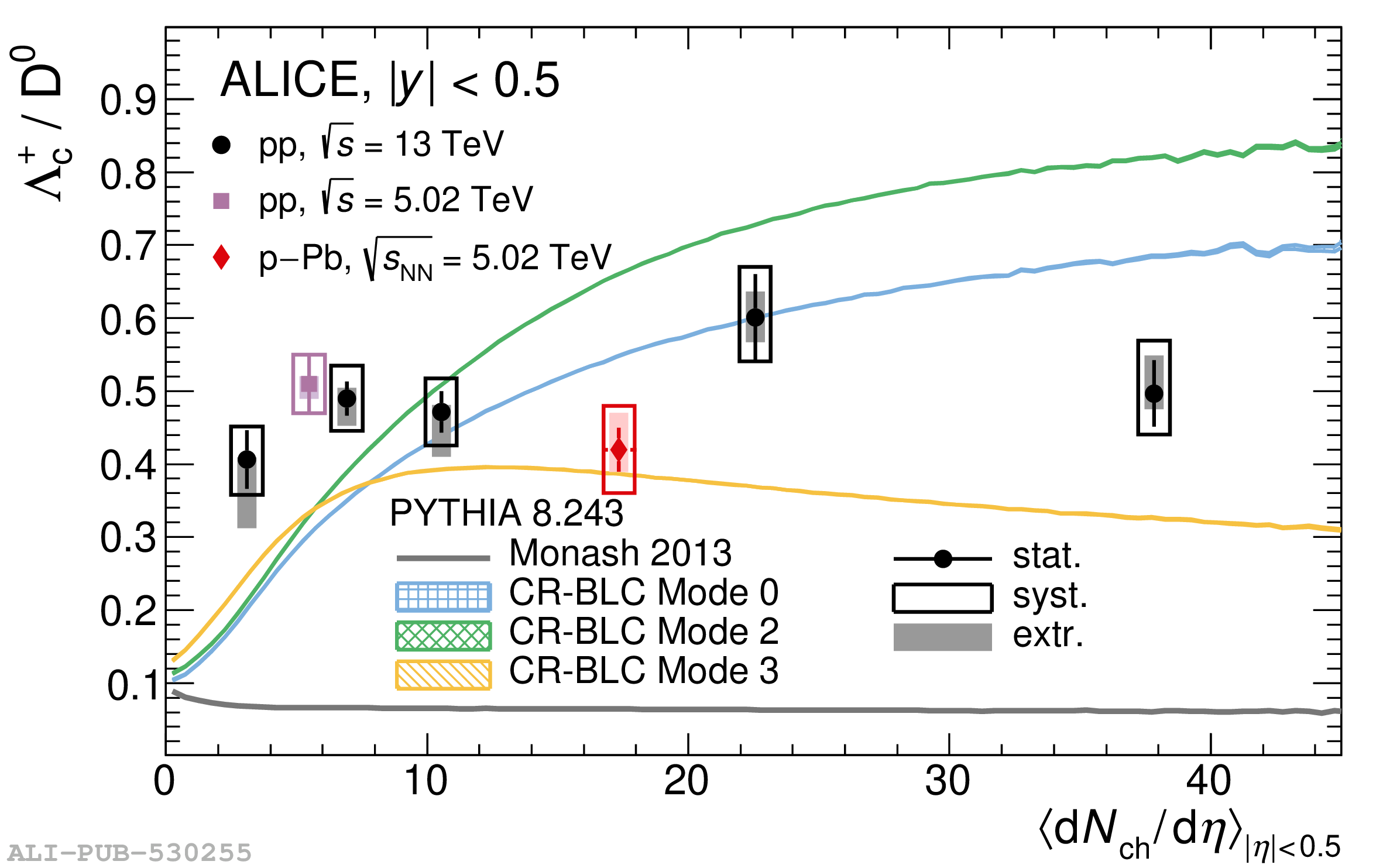# Figure 5

 Ratios of $p_{\rm T}$-integrated yields of $\Lambda_{\rm c}^+$ and $\rm{D^0}$ hadrons as a function of $\langle {\rm d}N_{\rm ch}/{\rm d}\eta \rangle$ in pp collisions at $\sqrt{s}=13$ TeV. Measurements performed in pp and p--Pb collisions at $\sqrt{s_{\rm NN}}=5.02$ TeV from Ref. [] are also shown. Statistical and systematic uncertainties are shown by error bars and empty boxes, respectively. Shaded boxes represent the extrapolation uncertainties. The corresponding PYTHIA predictions  are also shown.